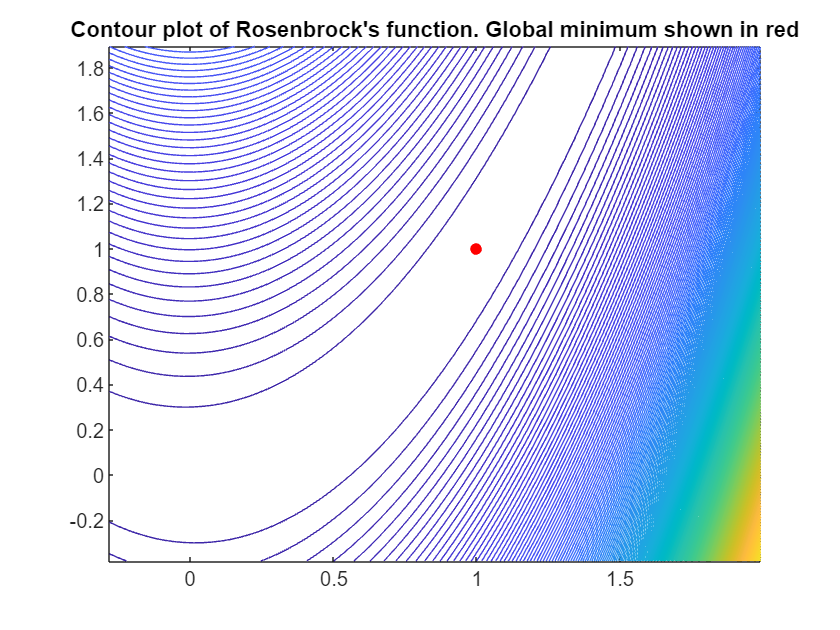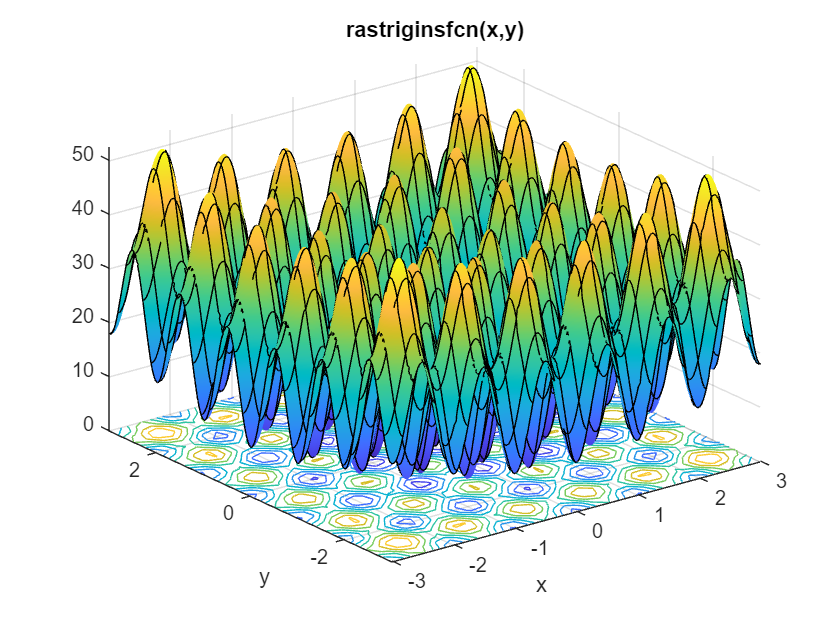# Which MATLAB Optimization functions can solve my problem?

The solvers function from Optimization toolbox is one of my favourite enhancements of R2022b because it helps improve my knowledge of which algorithms can solve my problems.

### Solving Rosenbrock with the default algorithm

Lets take the classic problem of minimising Rosenbrock's function as an example.
$f(x)=100\left ( x_2 - x_1^2\right )^2 + (1-x_1)^2$
The minimum is at (1,1) which is inside a long, narrow and flat valley. Many Optimization solvers find the valley rather easily but struggle with finding the global mimimum within it.
rosen = @(x,y) 100*(y - x.^2).^2 + (1 - x).^2;
fcontour(rosen,[-0.5,2.0],LevelStep=10)
hold on
scatter(1,1,300,'r.');
hold off
title("Contour plot of Rosenbrock's function. Global minimum shown in red")Using MATLAB's problem based Optimization workflow, we can solve this as follows
% Set up a couple of Optimization variables and apply boundaries
x = optimvar("x",LowerBound=-3,UpperBound=3);
y = optimvar("y",LowerBound = 0,UpperBound=9);

%Define the objective function
obj = 100*(y - x^2)^2 + (1 - x)^2;
%Set up the Optimization problem
prob = optimproblem(Objective=obj);

%choose a starting guess
x0.x = -2.1;
x0.y = 2.2;
% solve the problem
[sol,fval,exitflag,solution] = solve(prob,x0)
Solving problem using lsqnonlin. Local minimum possible. lsqnonlin stopped because the final change in the sum of squares relative to its initial value is less than the value of the function tolerance. <stopping criteria details>
sol = struct with fields:
x: 1.0000 y: 1.0000
fval = 4.9503e-16
exitflag =
FunctionChangeBelowTolerance
solution = struct with fields:
firstorderopt: 1.2147e-06 iterations: 26 funcCount: 27 cgiterations: 0 algorithm: 'trust-region-reflective' stepsize: 3.4494e-05 message: 'Local minimum possible.↵↵lsqnonlin stopped because the final change in the sum of squares relative to ↵its initial value is less than the value of the function tolerance.↵↵<stopping criteria details>↵↵Optimization stopped because the relative sum of squares (r) is changing↵by less than options.FunctionTolerance = 1.000000e-06.' objectivederivative: "forward-AD" solver: 'lsqnonlin'
MATLAB analysed the problem and decided to use lsqnonlin as the solver. This turned out rather well for us as it went on to find the minimum without any difficulty. There were only 27 function evaluations.

### What other algorithms could solve this problem?

You might ask yourself, however, out of all of the Optimization solvers in both Optimization Toolbox and Global Optimization Toolbox, which of them are capable of solving this problem as well? This is what the solvers function does for us. Give it an Optimization problem and it will return the default solver that MATLAB would choose for that problem.
[autosolver,allsolvers] = solvers(prob);
fprintf("The default solver for this problem is %s\n",autosolver)
The default solver for this problem is lsqnonlin
It also returns all other solvers that could tackle the input problem.
allsolvers'
ans = 10×1 string
"lsqnonlin"
"lsqcurvefit"
"fmincon"
"ga"
"patternsea…
"surrogateopt"
"particlesw…
"simulannea…
"gamultiobj"
"paretosearch"
Let's explicity instruct MATLAB to use one of these: fmincon
[sol,fval,exitflag,solution] = solve(prob,x0,Solver="fmincon")
Solving problem using fmincon. Local minimum found that satisfies the constraints. Optimization completed because the objective function is non-decreasing in feasible directions, to within the value of the optimality tolerance, and constraints are satisfied to within the value of the constraint tolerance. <stopping criteria details>
sol = struct with fields:
x: 1.0000 y: 1.0000
fval = 1.9355e-13
exitflag =
OptimalSolution
solution = struct with fields:
iterations: 46 funcCount: 71 constrviolation: 0 stepsize: 7.3444e-05 algorithm: 'interior-point' firstorderopt: 9.0385e-07 cgiterations: 14 message: 'Local minimum found that satisfies the constraints.↵↵Optimization completed because the objective function is non-decreasing in ↵feasible directions, to within the value of the optimality tolerance,↵and constraints are satisfied to within the value of the constraint tolerance.↵↵<stopping criteria details>↵↵Optimization completed: The relative first-order optimality measure, 9.038468e-07,↵is less than options.OptimalityTolerance = 1.000000e-06, and the relative maximum constraint↵violation, 0.000000e+00, is less than options.ConstraintTolerance = 1.000000e-06.' bestfeasible: [1×1 struct] objectivederivative: "forward-AD" constraintderivative: "auto" solver: 'fmincon'
So fmincon can and did solve the problem but it had to work much harder with 71 function evaluations. Just because the solvers function determines that an algorithm is capable of tackling a problem doesn't necessarily mean its a good fit. An example of a really bad fit for this problem is Simulated Annealing.
[sol,fval,exitflag,solution]= solve(prob,x0,Solver="simulannealbnd")
Solving problem using simulannealbnd. Optimization terminated: change in best function value less than options.FunctionTolerance.
sol = struct with fields:
x: 2.8612 y: 8.1900
fval = 3.4653
exitflag =
SolverConvergedSuccessfully
solution = struct with fields:
iterations: 2247 funccount: 2262 message: 'Optimization terminated: change in best function value less than options.FunctionTolerance.' rngstate: [1×1 struct] problemtype: 'boundconstraints' temperature: [2×1 double] totaltime: 0.2231 solver: 'simulannealbnd'
Yikes! That's rather far from the actual solution and we've already burned though over a thousand function evaluations. Perhaps simulated annealing isn't such a good idea for this function after all.
Let's try every solver that's valid for this problem using default settings for each one
variableNames = ["x solution","y solution","objective value","function evaluations"];
results = array2table(NaN(numel(allsolvers), numel(variableNames)));
results.Properties.VariableNames = variableNames;
results.Properties.RowNames = allsolvers;

for solverNum = 1:numel(allsolvers)
solver = allsolvers(solverNum);
% I want each solver to be quiet while it works.
options=optimoptions(solver,Display="None");
if solver=="surrogateopt"
options=optimoptions(options,PlotFcn=[]);
end
[sol,fval,exitflag,solution] = solve(prob,x0,Solver=solver,Options=options);
%Some solvers have solution.funcCount, others have solution.funccount
if isfield(solution,'funcCount')
funcount = solution.funcCount;
else
funcount = solution.funccount;
end
results.("x solution")(solverNum) = sol.x;
results.("y solution")(solverNum) = sol.y;
results.("objective value")(solverNum) = fval;
results.("function evaluations")(solverNum) = funcount;
end
results
results = 10×4 table
x solutiony solutionobjective valuefunction evaluations
1 lsqnonlin11027
2 lsqcurvefit11027
3 fmincon11071
4 ga0.94430.89040.00339453
5 patternsearch1.86583.48520.7511734
6 surrogateopt0.98850.97680.0001200
7 particleswarm0.99960.999305200
8 simulannealbnd2.34235.48831.80212151
9 gamultiobj1.01211.02440.00015100
10 paretosearch0.94970.90880.00749000
It seems that MATLAB's default choice for this problem, lsqnonlin, was a good one. Indeed it was the best one. That's not always the case though.

### Which algorithm is best for Rastigrin's function?

Consider Rastigrin's function. This is another classic test function in numerical optimization with many local minima and a global minimum at (0,0):
ras = @(x, y) 20 + x.^2 + y.^2 - 10*(cos(2*pi*x) + cos(2*pi*y));
Plotting it shows just how many local minima there are
fsurf(ras,[-3 3],"ShowContours","on")
title("rastriginsfcn(x,y)")
xlabel("x")
ylabel("y")Usually you don't know the location of the global minimum of your objective function. To show how the solvers look for a global solution, this example starts all the solvers around the point [2,3], which is far from the global minimum. It also uses finite bounds of -70 to 130 for each variable
x = optimvar("x","LowerBound",-70,"UpperBound",130);
y = optimvar("y","LowerBound",-70,"UpperBound",130);
prob = optimproblem("Objective",ras(x,y));
x0.x = 2;
x0.y = 3;
This time, MATLAB chooses fmincon as the default solver which gets stuck in one of the local minima
solve(prob,x0)
Solving problem using fmincon. Local minimum found that satisfies the constraints. Optimization completed because the objective function is non-decreasing in feasible directions, to within the value of the optimality tolerance, and constraints are satisfied to within the value of the constraint tolerance. <stopping criteria details>
ans = struct with fields:
x: -1.9899 y: -2.9849
As before, we use solvers to get the list of everything that could tackle this problem
[autosolver,allsolvers] = solvers(prob)
autosolver = "fmincon"
allsolvers = 1×8 string
"fmincon" "ga" "patternse… "surrogate… "particles… "simulannea… "gamultiobj" "paretosea…
Sweeping across all valid solvers again and we see that, this time, global Optimization functions such as patternsearch, particle swarm and simulated annealing do much better.
variableNames = ["x","y","objective value","function evaluations"];
results = array2table(NaN(numel(allsolvers), numel(variableNames)));
results.Properties.VariableNames = variableNames;
results.Properties.RowNames = allsolvers;

for solverNum = 1:numel(allsolvers)
solver = allsolvers(solverNum);
% I want each solver to be quiet while it works.
options=optimoptions(solver,Display="None");
if solver=="surrogateopt"
options=optimoptions(options,PlotFcn=[]);
end
[sol,fval,exitflag,solution] = solve(prob,x0,Solver=solver,Options=options);
%Some solvers have solution.funcCount, others have solution.funccount
if isfield(solution,'funcCount')
funcount = solution.funcCount;
else
funcount = solution.funccount;
end
results.("x")(solverNum) = sol.x;
results.("y")(solverNum) = sol.y;
results.("objective value")(solverNum) = fval;
results.("function evaluations")(solverNum) = funcount;
end
results
results = 8×4 table
xyobjective valuefunction evaluations
1 fmincon-1.9899-2.984912.934439
2 ga1.98992.984912.93443343
3 patternsearch00099
4 surrogateopt-0.99820.00941.0146200
5 particleswarm0.995000.99501500
6 simulannealbnd-0002864
7 gamultiobj-0005100
8 paretosearch-0.995000.99507153
It's worth bearing in mind that these 'solver sweeps' only consider the default settings for each solver. Each solver has a range of options that can improve performance and solution quality that haven't been considered here but we have made a good start in exploring the algorithms we can bring to bear on our problem.